# Posts Tagged ‘ Numerical Analysis ’

## Linearly spaced vectors in SAS

September 17, 2018
By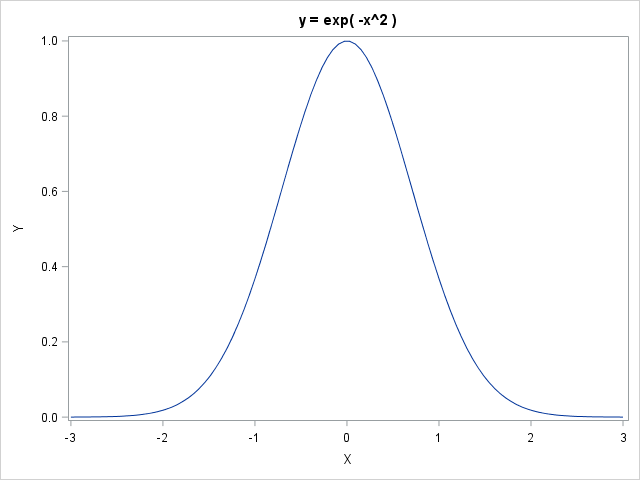The SAS/IML language and the MATLAB language are similar. Both provide a natural syntax for performing high-level computations on vectors and matrices, including basic linear algebra subroutines. Sometimes a SAS programmer will convert an algorithm from MATLAB into SAS/IML. Because the languages are not identical, I am sometimes asked, "what

Tags: , ,
Posted in SAS | Comments Off on Linearly spaced vectors in SAS

## Compute derivatives for nonparametric regression models

July 5, 2018
By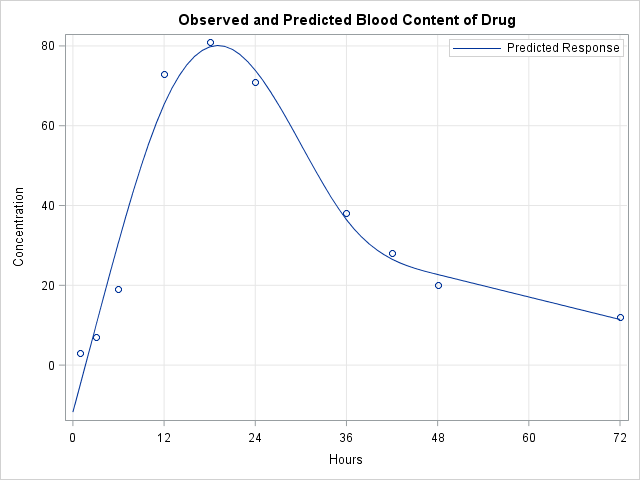SAS enables you to evaluate a regression model at any location within the range of the data. However, sometimes you might be interested in how the predicted response is increasing or decreasing at specified locations. You can use finite differences to compute the slope (first derivative) of a regression model.

Tags: , ,
Posted in SAS | Comments Off on Compute derivatives for nonparametric regression models

## Fit a distribution from quantiles

March 7, 2018
By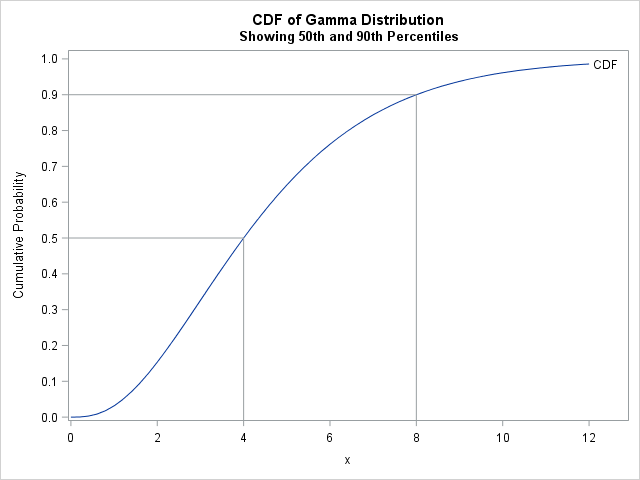Data analysts often fit a probability distribution to data. When you have access to the data, a common technique is to use maximum likelihood estimation (MLE) to compute the parameters of a distribution that are "most likely" to have produced the observed data. However, how can you fit a distribution

Tags: , , ,
Posted in SAS | Comments Off on Fit a distribution from quantiles

## Solve a system of nonlinear equations with SAS

February 28, 2018
By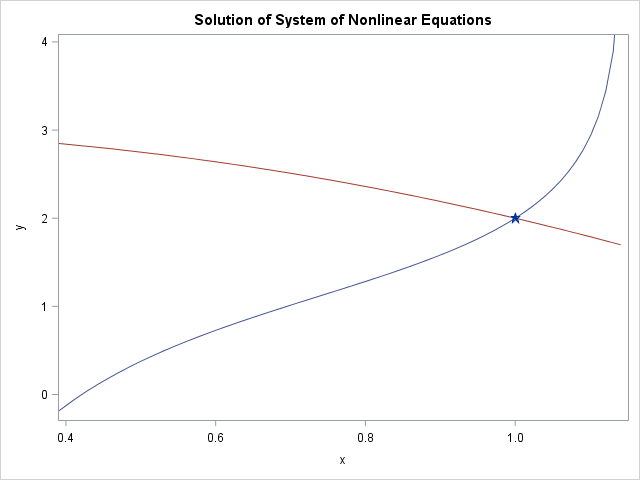This article shows how to use SAS to solve a system of nonlinear equations. When there are n unknowns and n equations, this problem is equivalent to finding a multivariate root of a vector-valued function F(x) = 0 because you can always write the system as f1(x1, x2, ..., xn)

Tags: ,
Posted in SAS | Comments Off on Solve a system of nonlinear equations with SAS

## Compute the quantiles of any distribution

February 19, 2018
By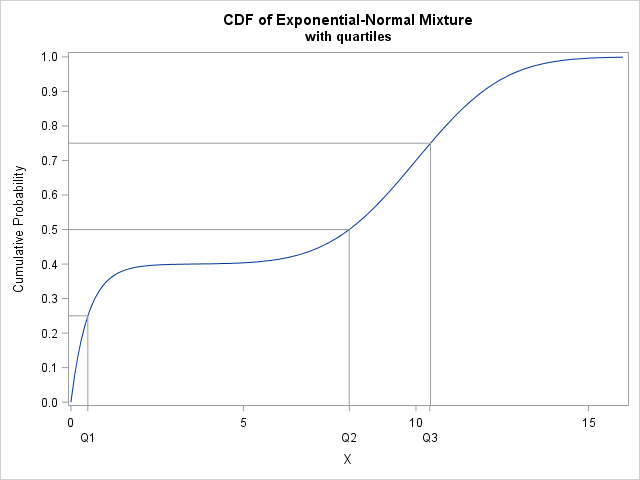Your statistical software probably provides a function that computes quantiles of common probability distributions such as the normal, exponential, and beta distributions. Because there are infinitely many probability distributions, you might encounter a distribution for which a built-in quantile function is not implemented. No problem! This article shows how to

Tags: , ,
Posted in SAS | Comments Off on Compute the quantiles of any distribution

## Simulate data from the beta-binomial distribution in SAS

November 20, 2017
By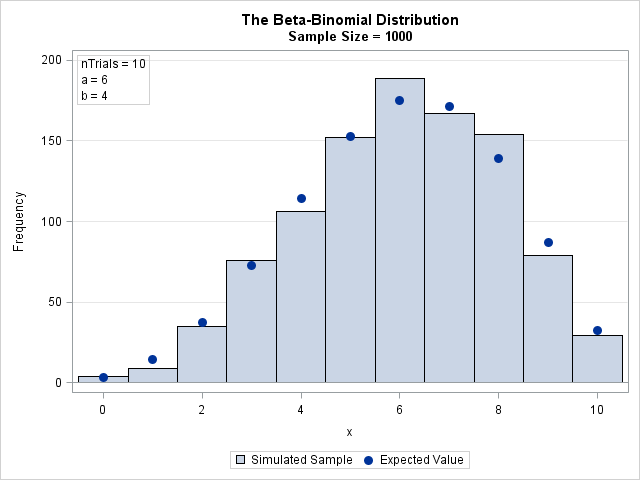This article shows how to simulate beta-binomial data in SAS and how to compute the density function (PDF). The beta-binomial distribution is a discrete compound distribution. The "binomial" part of the name means that the discrete random variable X follows a binomial distribution with parameters N (number of trials) and

Tags: , ,
Posted in SAS | Comments Off on Simulate data from the beta-binomial distribution in SAS

## Symbolic derivatives in SAS

September 11, 2017
By

Did you know that you can get SAS to compute symbolic (analytical) derivatives of simple functions, including applying the product rule, quotient rule, and chain rule? SAS can form the symbolic derivatives of single-variable functions and partial derivatives of multivariable functions. Furthermore, the derivatives are output in a form that

Tags: , ,
Posted in SAS | Comments Off on Symbolic derivatives in SAS

## The singular value decomposition: A fundamental technique in multivariate data analysis

August 28, 2017
By

The singular value decomposition (SVD) could be called the "billion-dollar algorithm" since it provides the mathematical basis for many modern algorithms in data science, including text mining, recommender systems (think Netflix and Amazon), image processing, and classification problems. Although the SVD was mathematically discovered in the late 1800s, computers have

Tags: ,
Posted in SAS | Comments Off on The singular value decomposition: A fundamental technique in multivariate data analysis

## Welcome!

SAS-X.com offers news and tutorials about the various SAS® software packages, contributed by bloggers. You are welcome to subscribe to e-mail updates, or add your SAS-blog to the site.Dear readers, proc-x is looking for sponsors who would be willing to support the site in exchange for banner ads in the right sidebar of the site. If you are interested, please e-mail me at: tal.galili@gmail.com